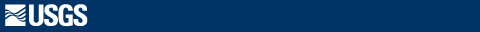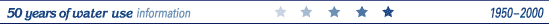Estimated Use of Water in the United States in 2000

Conversion Factors

Multiply By To Obtain
Area
acre 43,560 square foot (ft2)
4,047 square meter (m2)
0.001562 square mile (mi2)
Flow rate
gallon per day 3.785 liter per day
million gallons per day (Mgal/d) 1.121 thousand acre-feet per year
0.001547 thousand cubic feet per second
0.6944 thousand gallons per minute
0.003785 million cubic meters per day
1.3815 million cubic meters per year
billion gallons per day (Bgal/d) 1.3815 billion cubic meters per year
thousand acre-feet per year 0.8921 million gallons per day
0.001380 thousand cubic feet per second
0.6195 thousand gallons per minute
0.003377 million cubic meters per day

Some water relations in inch-pounds units are shown below:

1 gallon (gal) 1 million gallons (Mgal) = 8.34 pounds = 3.07 acre-feet = 62.4 pounds = 7.48 gallons = 325,851 gallons = 43,560 cubic feet = 17.4 million gallons per square mile = 27,200 gallons per acre = 100 tons per acre